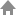# A-level微积分之函数凹凸性

2019-09-09 | 编辑：y | 分享到

Concavity

Let f be differentiable on an open interval I. If f' is increasing on I, then f is concave up on I. If f' is decreasing on I, then f is concave down on I.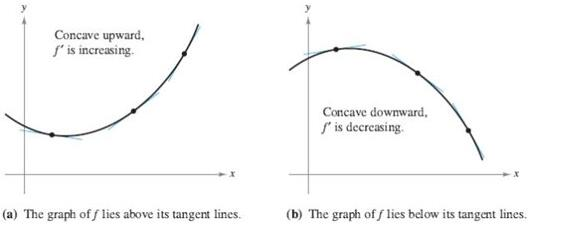The Second derivative and Concavity

Suppose that f'' exists on an open interval I.

• If f'' >0 on I, then f is concave up on I.

• If f'' < 0 on I, then f is concave down on I.

• If c is a point of I at which f'' changes sign at c (from positive to negative, or vice versa), then f has an inflection point at c.

• 如果在区间内 f'' > 0 on I,则函数图像向上凹（cancave up）；

• 如果在区间内 f'' < 0 on I,则函数图像向上凸（cancave down）；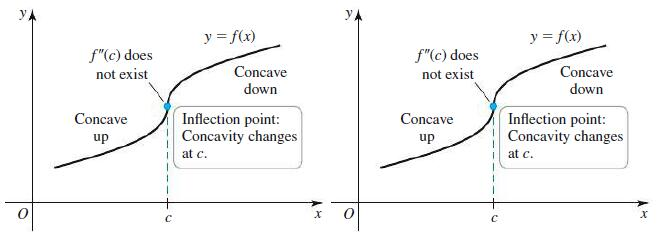Second Derivative Test

We need to use the second derivative to identify local maxima and minima.

Second Derivative Test for Local Extrema

Suppose that f'' is continuous on an open interval containing c with f' ( c)=0.

• If f'' > 0, then f has a local minimum at c .

• If f'' < 0, then f has a local maximum at c .

• If f'' = 0, then the test is inconclusive; f may have a local maximum, local minimum, or neither at c.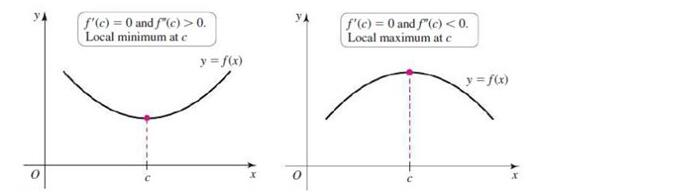Example 1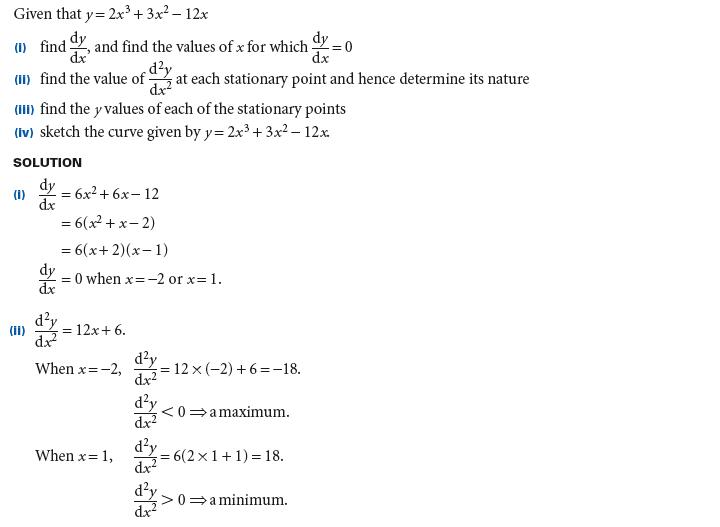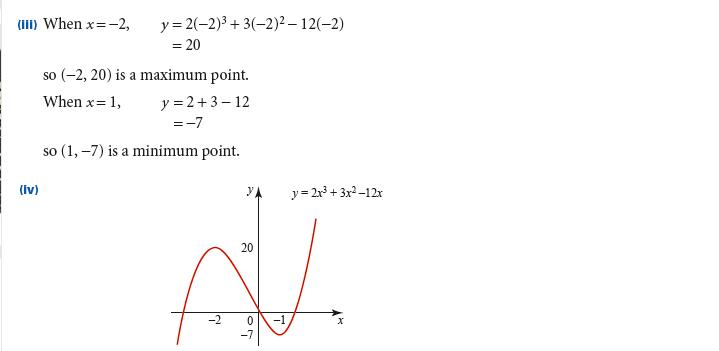一阶导数测试与二阶导数测试的目的是一样的，确定函数的相对极值性质，是最大值还是最小值。在A-Level考试中，使用一阶导数测试还是二级导数测试，取决于斜率为零的关键点的个数与函数二次求导的复杂程度。如果一阶导数为零的点较多，大于等于三个，建议使用二阶导数测试，如果阶导数为零的点只有一个或两个，且二阶导数求导复杂，可使用一阶导数测试。

 上一篇:Alevel经济备考：通货膨胀问答题怎么解决 下一篇:A-Level是什么意思？你了解它吗？1997年环球教育，2006年获得软银赛富2500万美元投资。2010年美国纳斯达克上市。0元模考| 机经下载• 李开云 |
• 郑睿 |
• 傅雪芬 |
• 李洁 |
• 唐巧 |
• 李荣华 |